# Completing the Square

### Brian McLogan

Why does completion square have a terrible reputation? Why do students dislike filling out squares? I remember completing the square a lot when I first started teaching, and students would say, “I understand math, but the one thing I don’t remember is completing the square.”

Completing the square is one of those processes that elude me. And I believe one of the reasons we struggle with completing the square is that students don’t recognize the relationships we’re looking for, which will be useful to apply to a quadratic equation in this video. What I want to do is investigate these connections. As a result, you better understand what completing the square entails and why we want to do it.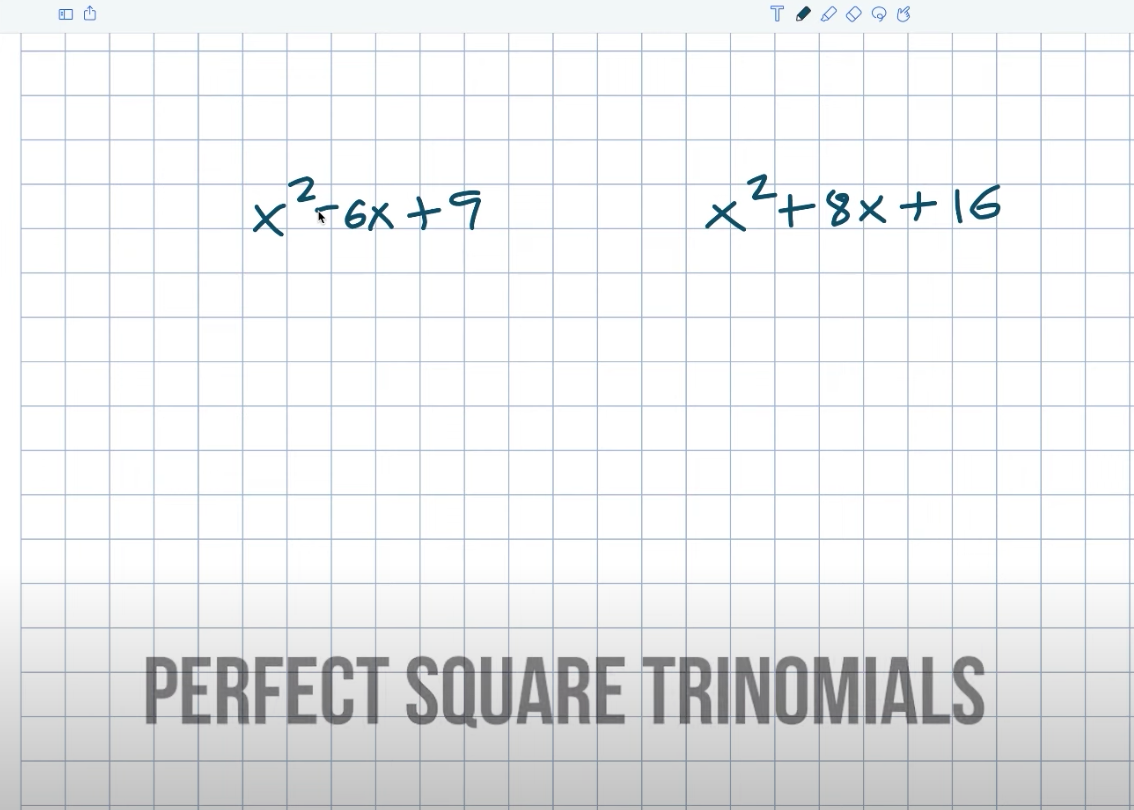^ Watch the full tutorial by clicking the photo above ^

## Example #1

So let’s begin with these two examples. You might notice these two quadratics and think, “Hey, I remember these.” Yes, these are what are known as perfect square trinomials. We call them perfect square trinomials because the first term can be written as a square number, and the last term can also be written as a square number. Again, this is something I want you to understand. The fact that the first and last terms are squared numbers does not imply that the triangle is a perfect square triangle. My middle term must be two times the square root of this last number in order for it to be a perfect square trinomial. So what I recognize here is that six is two times three.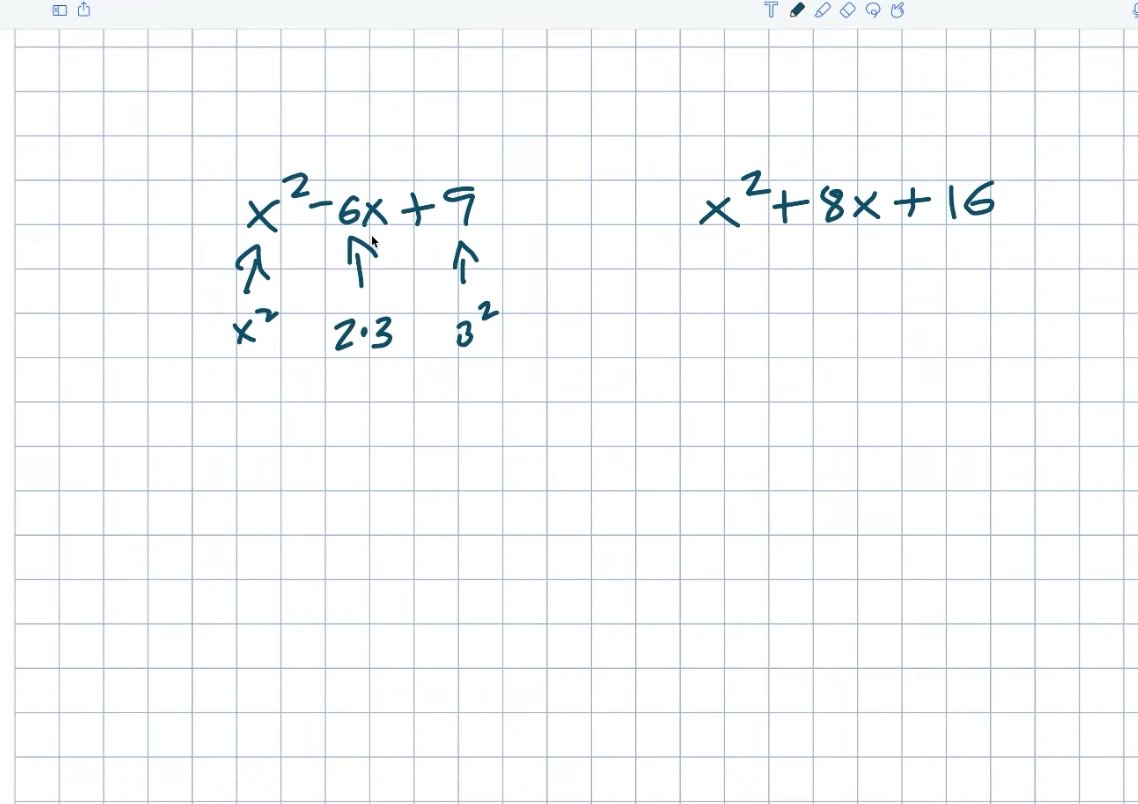Don’t even think about the negative for a second. We’ll get to that distinction in a moment. Let’s take a look at this example now. And, once again, we can see that this is an X squared. That is an X time X squared quantity. And this one will be four times four numbers squared. And you’ll notice that the middle will be two times four. Recognizing this relationship is crucial right now. And it’s crucial because this is exactly what we want to achieve when attempting to complete the square.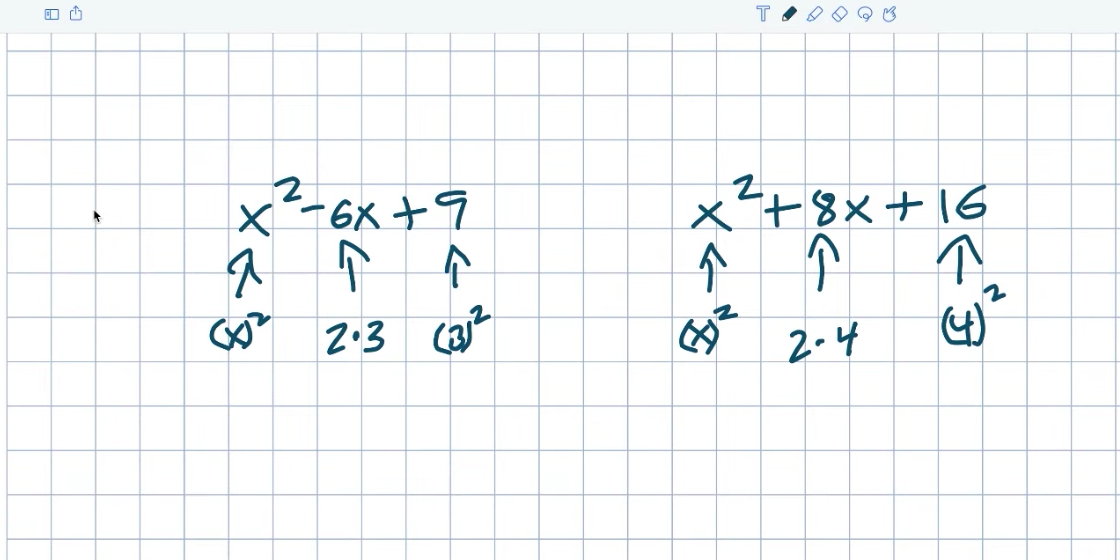Creating a perfect square Trinomal is what truly completes the square. And the reason these are so special is that when we first learn how to factor, it can be difficult. However, because we have a perfect square trinomial, we can factor it as a binomial square. Don’t worry if you’re not sure if you trust me on this one yet. As I recall, X minus three quantity squared is X minus three times an X minus three. Again, if you simply want to double-check your work, you can always multiply everything back out. Right? As a result, X times X equals X squared. Negative three times X equals a negative three X, negative three times X equals a negative three X, and negative three times negative three equal a positive nine. So, once again, we’re getting this X squared, we’re getting the nine, and you can see how there are two threes with the negative three, X minus X. Again, all the really negative is going to tell us is what the sign in your binomial will be. We’re going to get the same result in this example.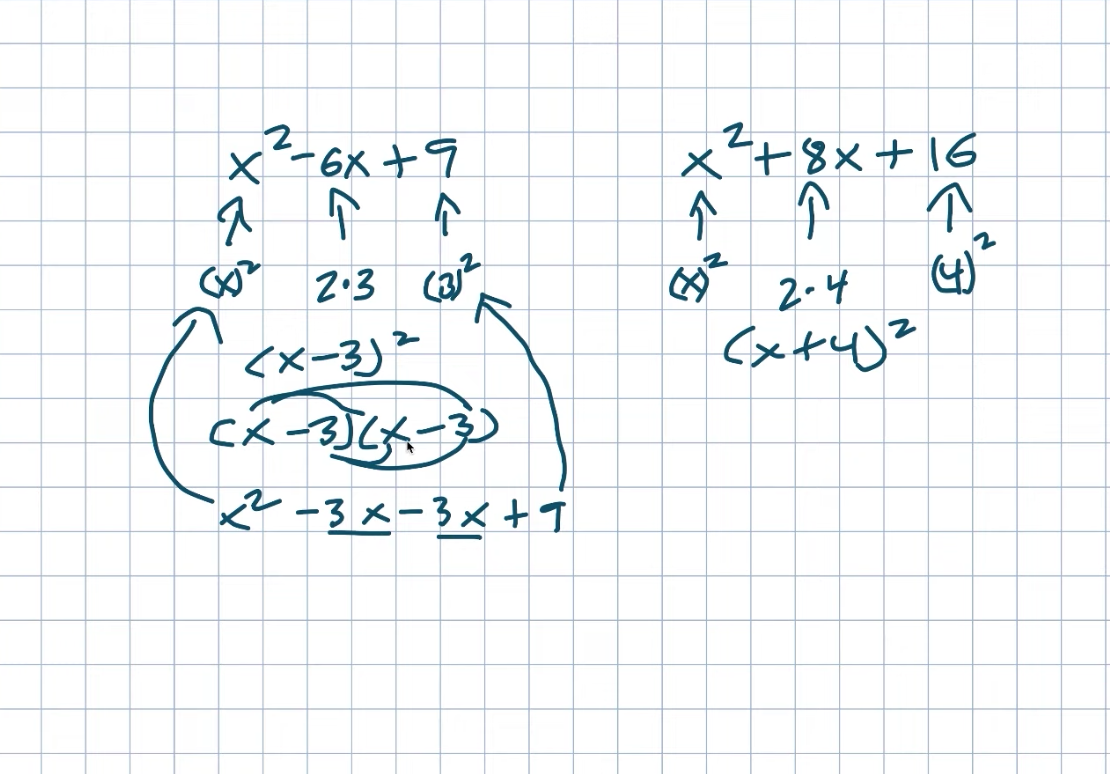And if you want to expand it and look at my work, you are welcome to do so. However, you should be able to see that when we have a perfect square triangle mail, we can easily factor these down to a binomial squared. And I want you to remember this relationship between my first, middle, and last terms because we’re going to use it to solve some problems in this video.

## Example #2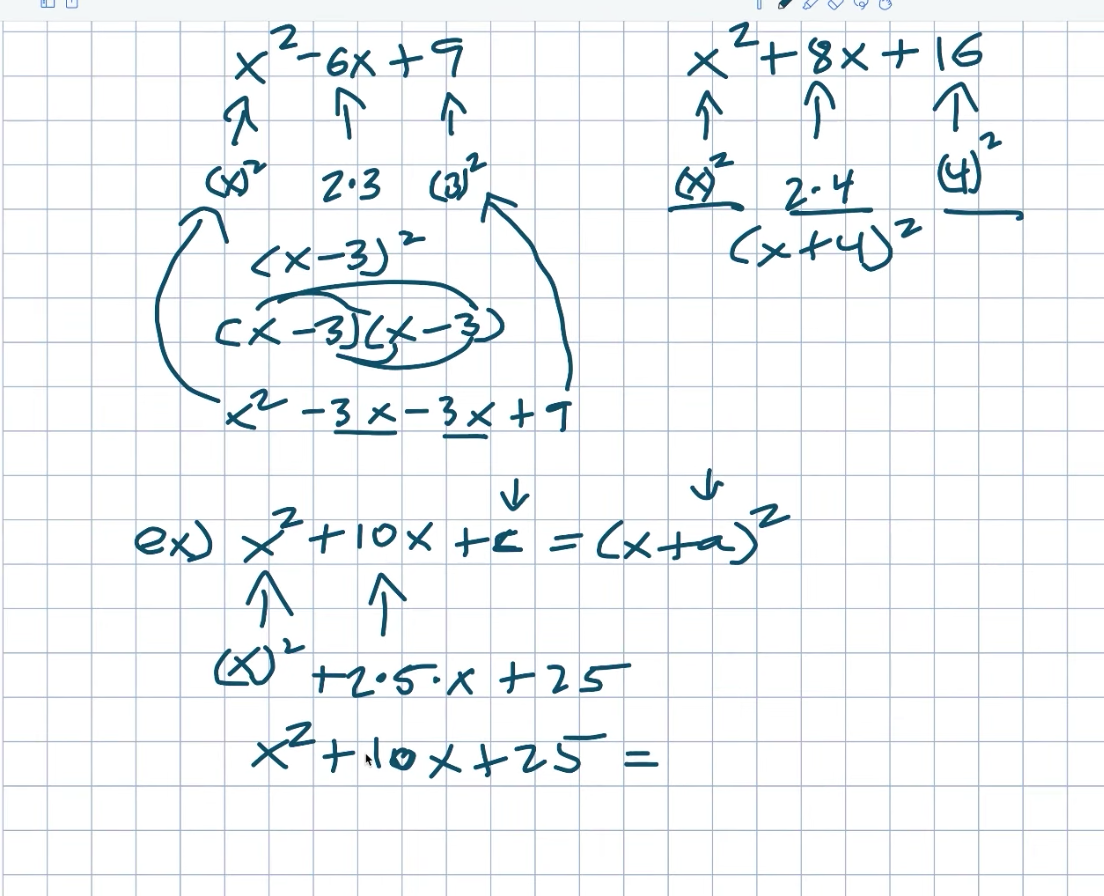So, let’s look at this first example to see how we can identify what if we needed to find our value C and our value a. What exactly does this relationship with the perfect square trinomial have to do with identifying these missing values? Let’s pretend this is a perfect square trinomial. As a result, it can be factored to a binomial squared. Now recall the first term. Right. We already have an exquisite, so that will be good. We’ve already decided on that. If there is a perfect square trinomial, my middle term will be two times something. So, if I want to say two times, what will give me ten? And we all know the answer is going to be a five. Again, a simple way to always identify that value is to divide your middle term by two. That will always provide you with that value. To find C, remember that we will square this five, giving me a 25. As a result, this can be reduced to an X squared plus a ten, X plus 25, which is now my perfect square triangle. I want to know now what the factored form of this is. So you don’t need to know how to factor in this. Hopefully, you already recognize this, simply an X plus five quantities squared. But I want you to notice here that my C is being squared. Right. So really, all I have to do is take my five and square it. That’s how I arrived at 25, which eventually became my C value. If you recall from my previous video where I introduced completing the square, we discussed C being equal to an H squared. In this example, that’s similar to what’s going on, but we’re not using H. In this case, what C equals is now a B divided by two, correct? That’s exactly what I did. I divided ten by two to get five, and then I squared it. As a result, B divided by two squared equals C. Then, I want you to notice the relationship between C and A. And basically, if I take C, which is 25, and takes the square root of that number, I’ll get a five, which is exactly my value. Another way to look at this is that A is simply equal to a B divided by two. So, before you square it, divide B by two. This is how you can find your A.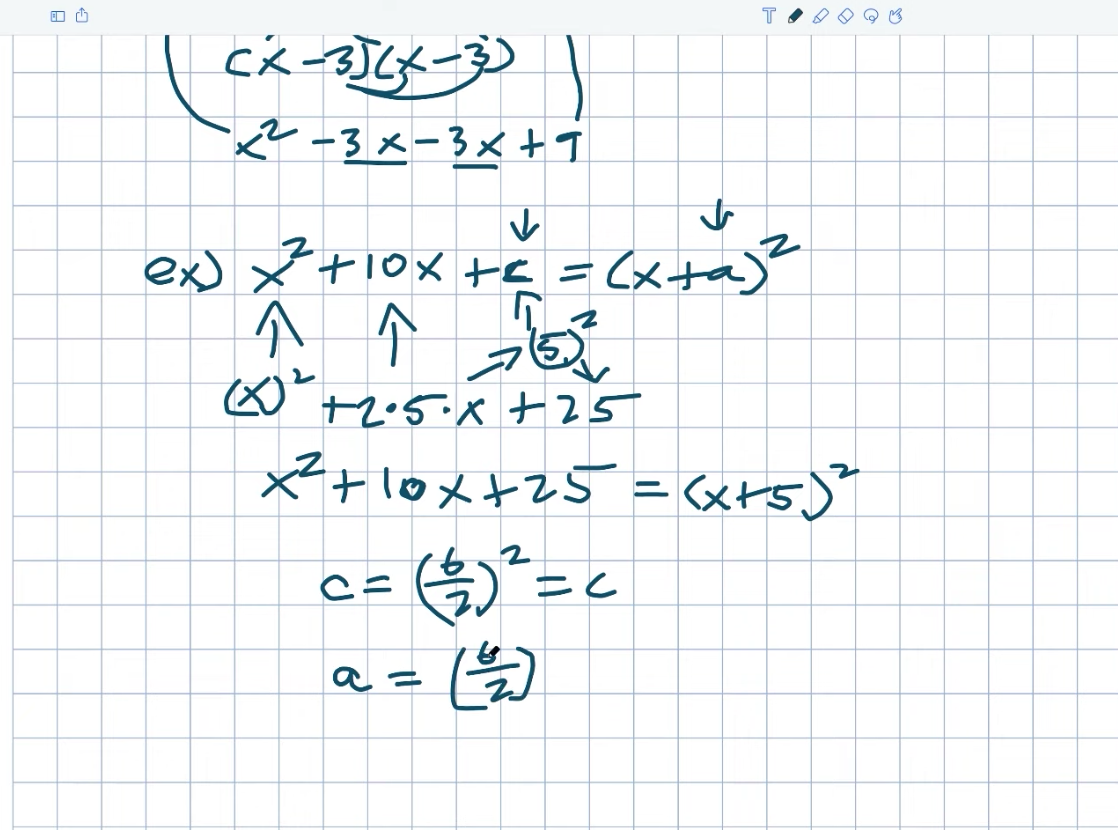So, let’s go ahead and apply these two rules to see if we can solve some more problems that may be a little more difficult. Okay. So, in this case, I can do the same thing. If I just want to follow the rules here, I’d like to find C. I can just say, “Well, that’ll be a B divided by two quant squared, right?” In this case, it will be 14 divided by two, which equals men’s quantity squared. As a result, 14 divided by two equals seven. Seven times seven equals 49. Let’s go figure out what my A is now. So, once again, remember that A equals B divided by two. However, not with the square. So, when I do this, I take a 14 and divide it by two, which gives me a seven. But, once again, it’s critical to match the sign of your binomial with the sign of your Trina. Again, it’s already been done for us in this case, but it’s important to acknowledge that fact. So, if I want to write it with my correct values, I can write it as X squared plus 14. X plus 49 equals an X plus seven numbers squared. When we start introducing fractions, students usually become a little confused. But that’s perfectly fine. Simply follow the rules that we have established here. Again, we’re looking for a C value that results in a perfect square Trinoma. Three cannot be written as two times any number, at least not with integers. So don’t worry, we’ll have fractions.

## Example #3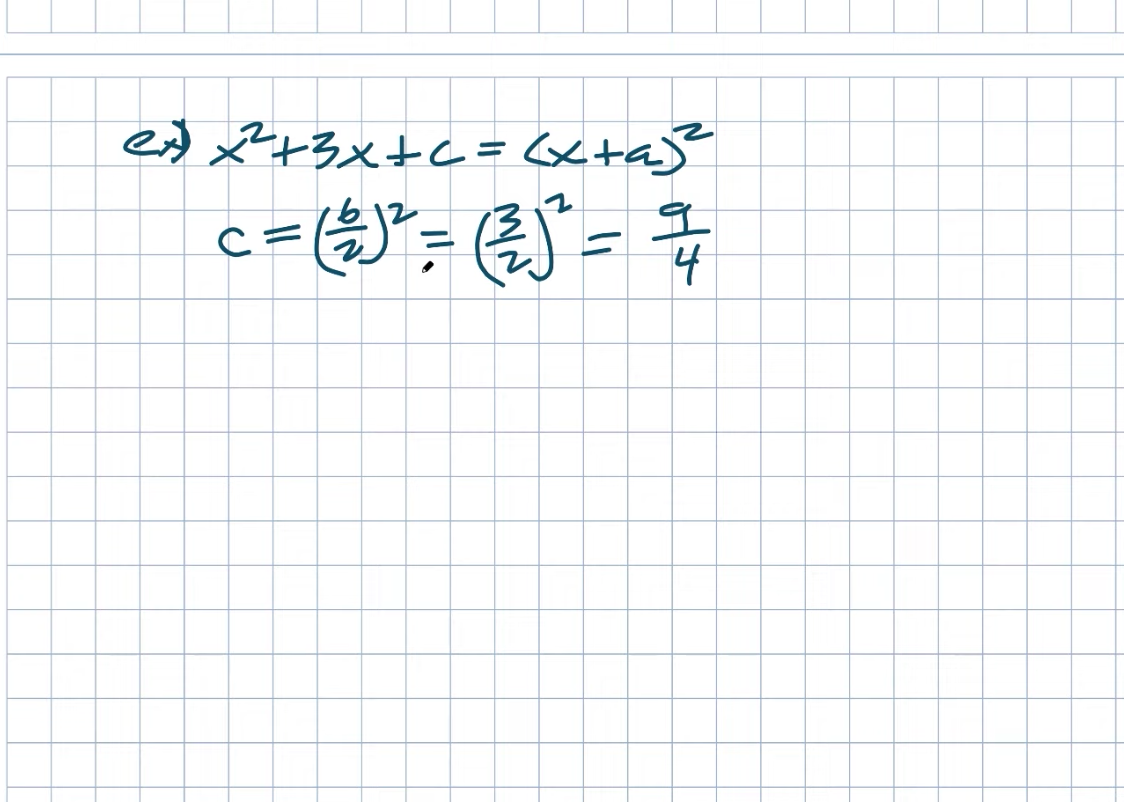So, remember that C is equal to B divided by two quantities squared because this relationship does not vanish. This number is created by multiplying two times the number if it is a perfect square trinomial and then squared. So, returning to this point, all I have to do is square a three divided by a two. I’m going to square the three and the two using the quotient of the product rule. That’ll give me a nine over four. Again, hopefully, you recognize that my A is just going to be a B over two, which is just going to be a three-hundredths. As a result, using these fractions, I can rewrite this as a perfect square trinomial and a binomial squared. And, once again, even if this appears to be a little confusing, it is important to recognize that it is important to recognize like let’s just actually expand this out just to make sure because a lot of times, students will like it once we get fractions, they just kind of forget everything that we may have learned. So I’m just going to multiply this out with you quickly so you can see exactly how this works, or at least a way for you to do a mental check for this.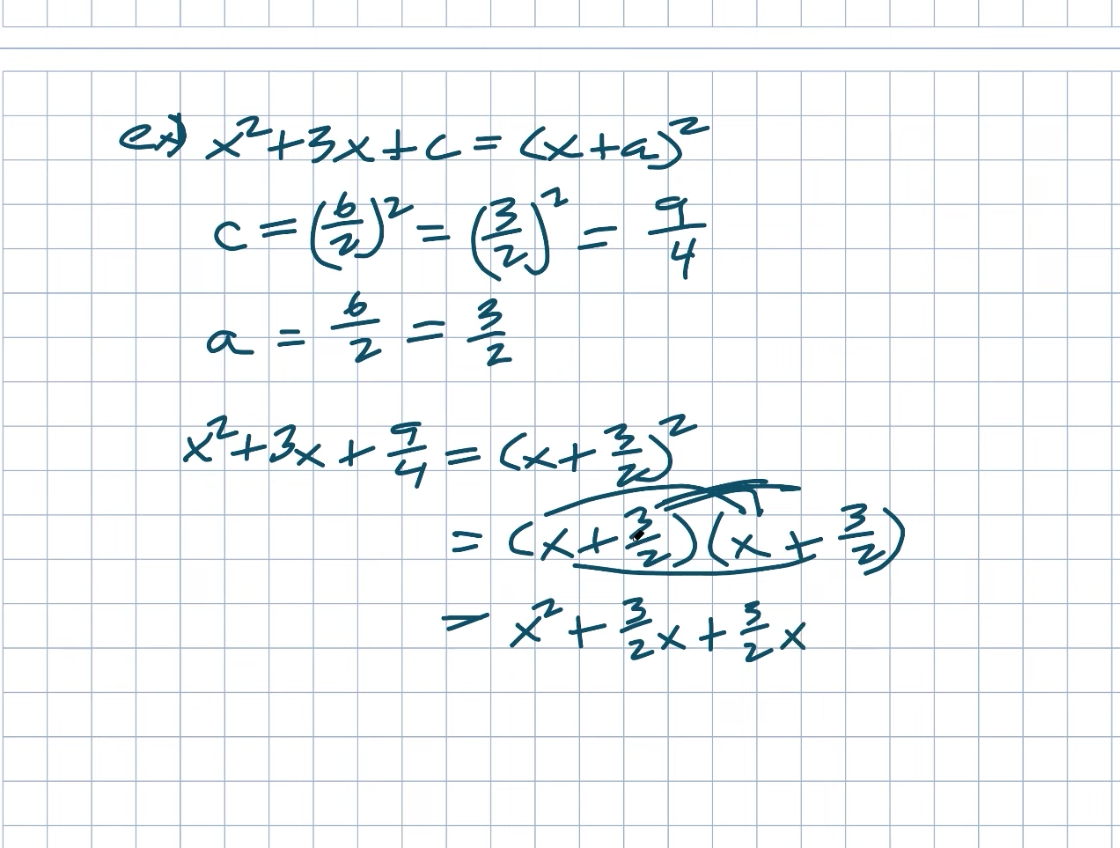So we know that when we multiply X by X, we get X squared, right? As a result, we’ll have a three-half X that will be with those two. And then we can do these two, three, two X plus a three, two X plus a three, two X plus a three, two X plus a three, two X plus a three, two X plus a three, two X plus a three, two times three When multiplying fractions, remember to multiply the numerator by the denominator. As a result, that equals a force of nine. I’m now adding these fractions together. They do, however, have the same numerator and denominator. Right. Because they share a common denominator, I can combine them. I’m not particularly concerned with the denominator. But, once again, what is going to be three plus three equals six over two? So, six over two equals a three X. As a result, I simplified this to an X squared plus a three X plus a nine over four.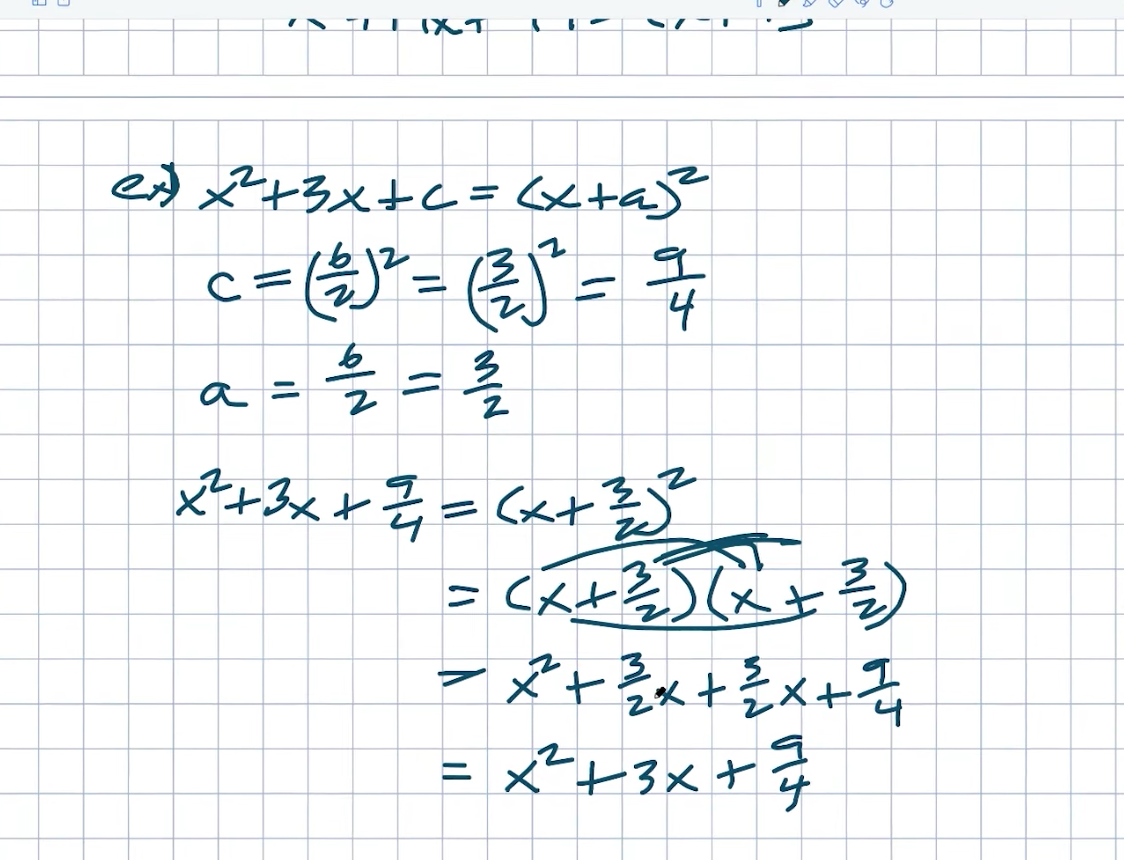If your teacher gives you fractions like this and you’re confused, remember that you can always check your work by multiplying it back out to step it up one more time. In this case, what if we begin with the middle term? Is that a fraction? Again, just stick to the rules. You can do it if you are confident in your ability to work with fractions. Remember that C is simply B divided by two quantities squared. Okay. So let’s take it one step at a time. B multiplied by the square of two numbers. Again, in this case, B, we’re not going to be concerned about the sign B equaling a positive one-two. So what I’m going to do is have a one-two divided by two squared quantities. How do you divide by two once more? That, once again, is what we need to figure out for a. Right. Remember that A is equal to one-half divided by two. So let’s start with a first. Remember that dividing by two is the same as multiplying by a reciprocal or multiplying by the reciprocal. So what I want to do is divide it by half. I want to do that because it will make the denominator equal to one, which, when divided by one, will be whatever the numerator is. As a result, two times one half equals one. As a result, in this case, I have a one-four divided by a one, correct? Which is the same as a one-four. So, when I divide one-half by two, I get a one-fourth quantity squared, which is equal to one over sixteen. Again, we don’t need to be concerned about the signs because they’ve already arrived, right? Again, notice that the binomial and trinomial must be the same size. So, if I just plug them in to see if it works, I get an X squared minus one two X plus one over 16 plus an X minus one four square. You can check my work by expanding that to see what I mean, as we did in the previous example. But, hopefully, you now have a little more confidence in determining the value of C as well as the value of a.

## Example #4Now, one last type of example that I really want to work on in this example to really ensure that you Hone your understanding of completing the square and the relationships between your first, middle, and the last term is what if we needed to find our middle term? And I’m sorry if my sixes appear to be BS. I had no idea I was doing it incorrectly until students called me out in class and said, hey, why are you writing those like that? What do you mean? I’m like, what do you mean? That’s how I always write my sixes. And they told me, “I guess I’m supposed to do it this way.” I’m not sure. I’ve always written them like this. And every now and then, a B appears to be a six. Alternatively, when I do, A-B-I does a B like that. So I’m not sure, sometimes they all look the same, but people sometimes call me out for whatever reason. So, let’s get back to business. How do I determine what B is? So, in the previous example, we figured out C, right? C was calculated by dividing B by two quantities squared. So this value is B divided by two quantities squared, correct? I’m attempting to decipher B. So let’s just go over this again. So four equals a B divided by two quantities squared. So, to solve this, I’ll simply take the square root of both sides. Again, introducing the square root will result in a plus or minus two, which equals a B divided by two. Now all I have to do is find my B because I’m just going to multiply by two on both sides. We need to figure out whether this plus or minus four will equal like; which one is it? And, when I look at this example again, it doesn’t really matter if you use a plus or minus B because both would work. Because this is already X squared minus ABX plus four, we’ll just use the positive version of the solution in this case. As a result, we can simply say that B will equal four, and we can rewrite this as X squared minus four, X plus four, which hopefully you recognize as a perfect square trinomial. Right. This can also be expressed as X minus two quanta squared. Take a look at how things are moving.

## Example #5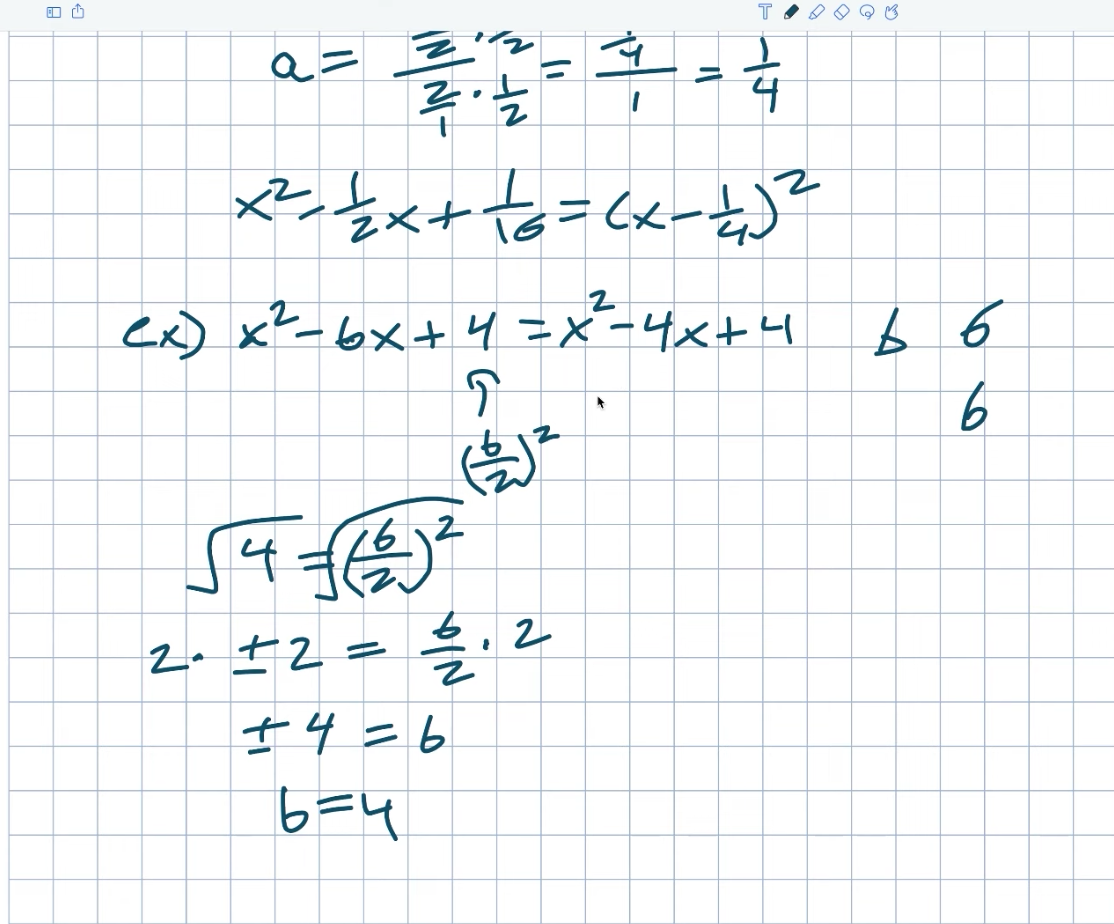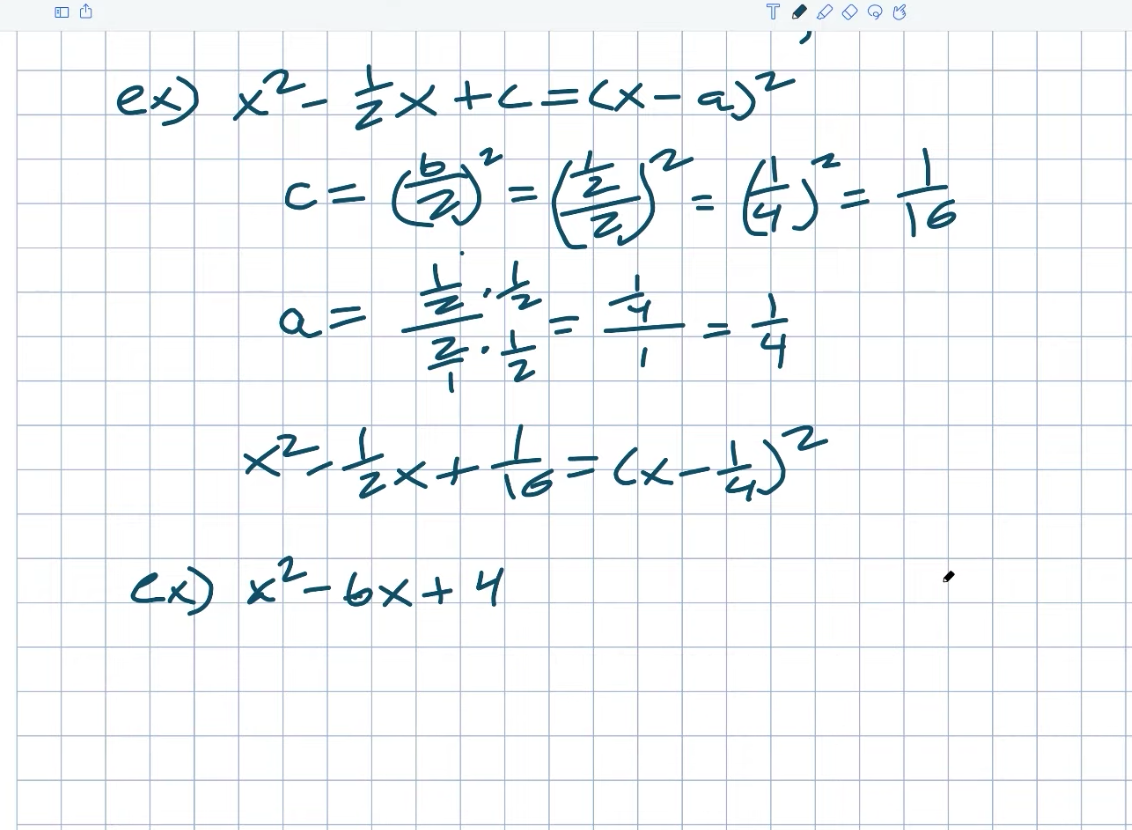Okay, let’s move on to another example. It becomes a little more difficult. So, in this example, you can see that my B will be a 16 over nine. So, once again, remember that our relationship C equals a B divided by two quantities squared. So, in this case, my C is a 16 over nine, which equals a B divided by two quantities squared. And that’s what we’re hoping to find. It’ll be our backup. The first thing I’ll do is take the square root of both sides, and again, the square root of the numerator and the square root of the denominator will be four third. You could use your plus or minus again, but it doesn’t really matter. We already know that we’re just trying to find our value of B, which could be positive or negative but is positive in this case. As a result, I’ll just leave it at that. As a result, that will equal a B divided by two. We must now solve for a B once more.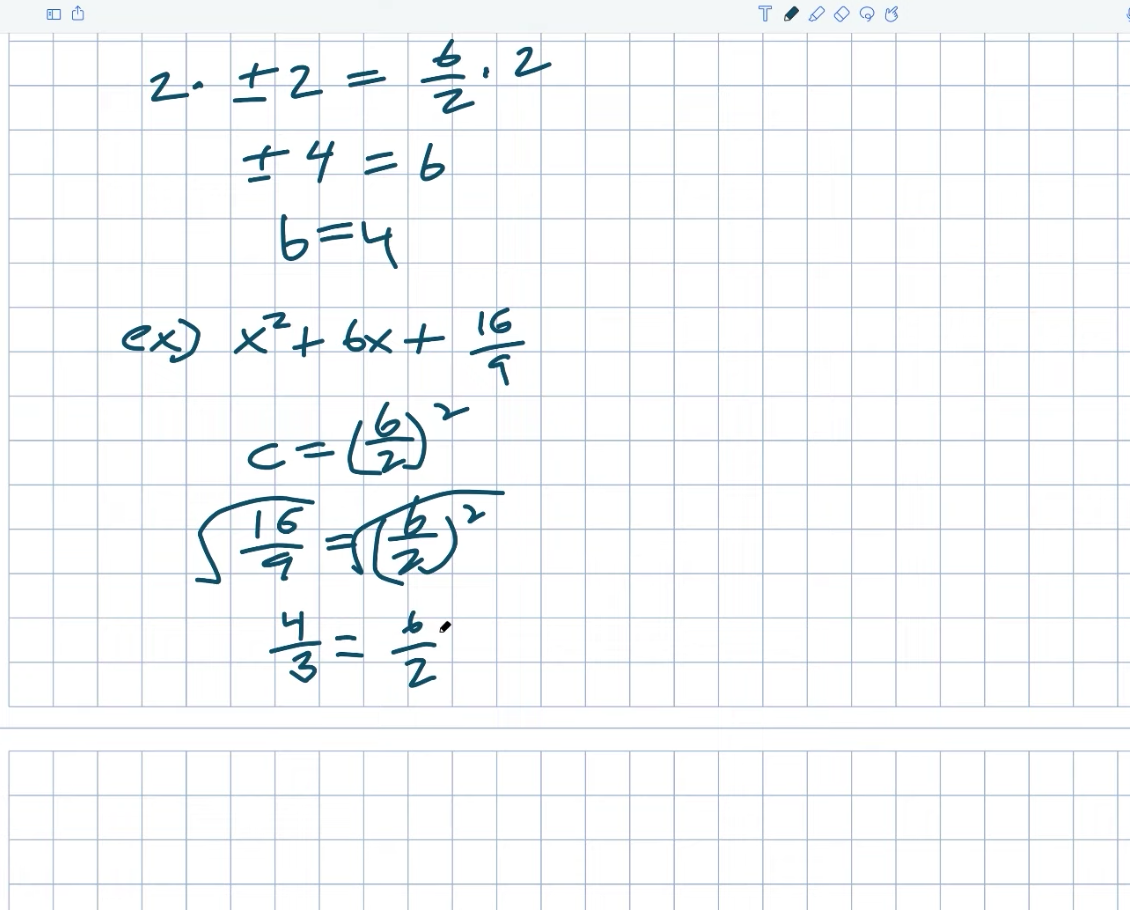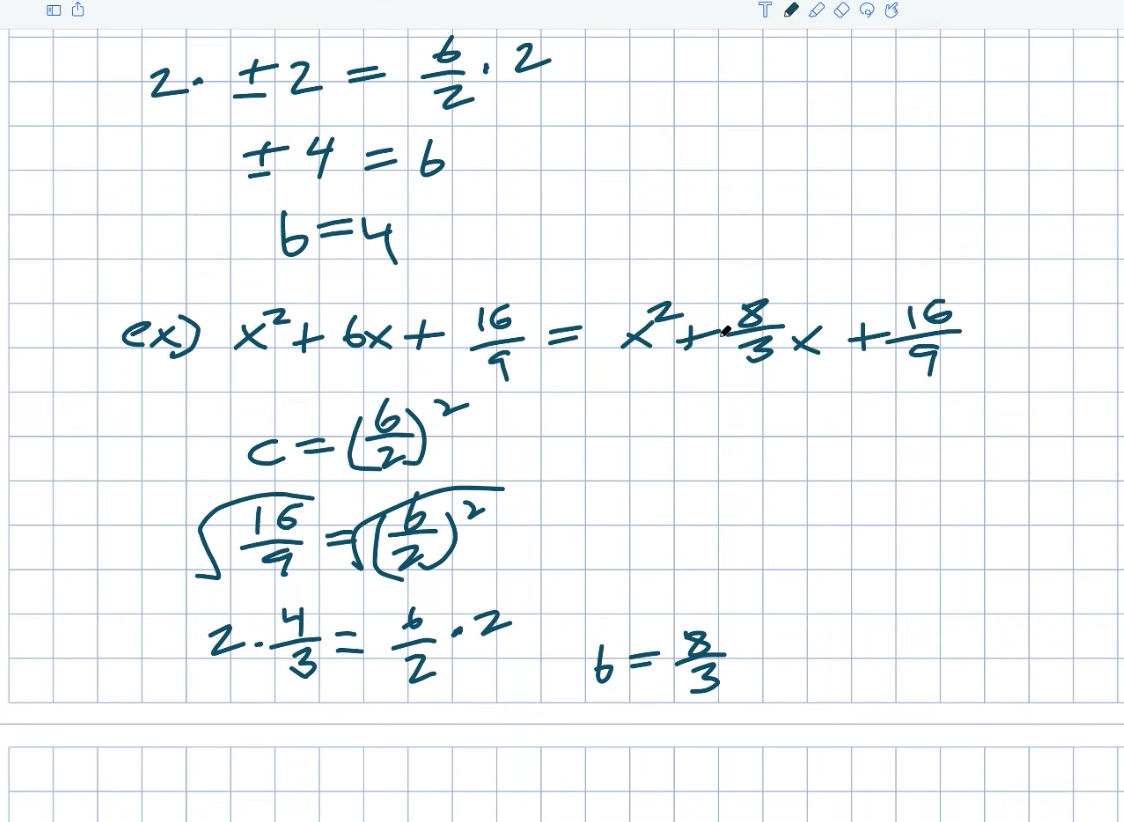Right. So I’ll multiply two on both sides, and the result will be a B equal to eight threes. X squared plus an eight three, X plus a sixteen over nine. Right. As a result, that will be my B value. And, once again, I hope you recognize that we can go ahead and factor this because, once again, what is our A, right? A is simply our B divided by two, which we have already determined. Guys, our B divided by two is four-thirds right here. So I could simplify this to an X plus a four-thirds quantity squared. And, once again, take a look at this work. Check to see if this works. Is it true that four-thirds times four-thirds equals sixteen nights? It does, indeed. Isn’t it true that four thirds plus four-thirds equal eight thirds? It does, indeed. Okay, fine.

## Example #6

Let’s have a little fun with this last example. We can get started on this one right away. This one will be a little more entertaining because 96 is not a square number. What is the significance of this? Remember that C equals B divided by two clients squared. So, what’s the first thing we’re going to do? We multiply both sides by the square root. So, how do I find the square root of 96? Remember that you want to break this down into a product with square numbers. So the largest square number I can think of that divides into 96 is 16 times six. As a result, I can divide this up. Now multiply 16 times the square root of six by the square root of the square root. The square root of 16 will be a four. So, all right, let’s just get this down on paper. So 96 is equal to B divided by two, quantity squared, correct?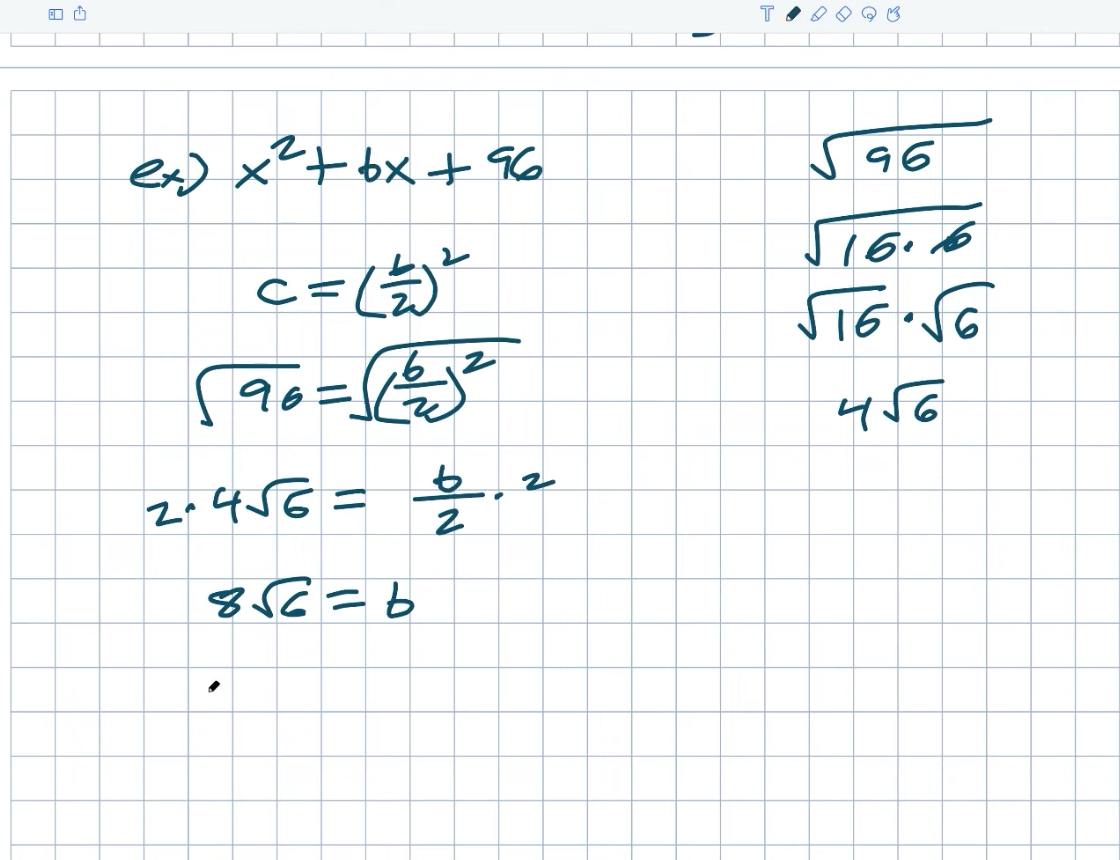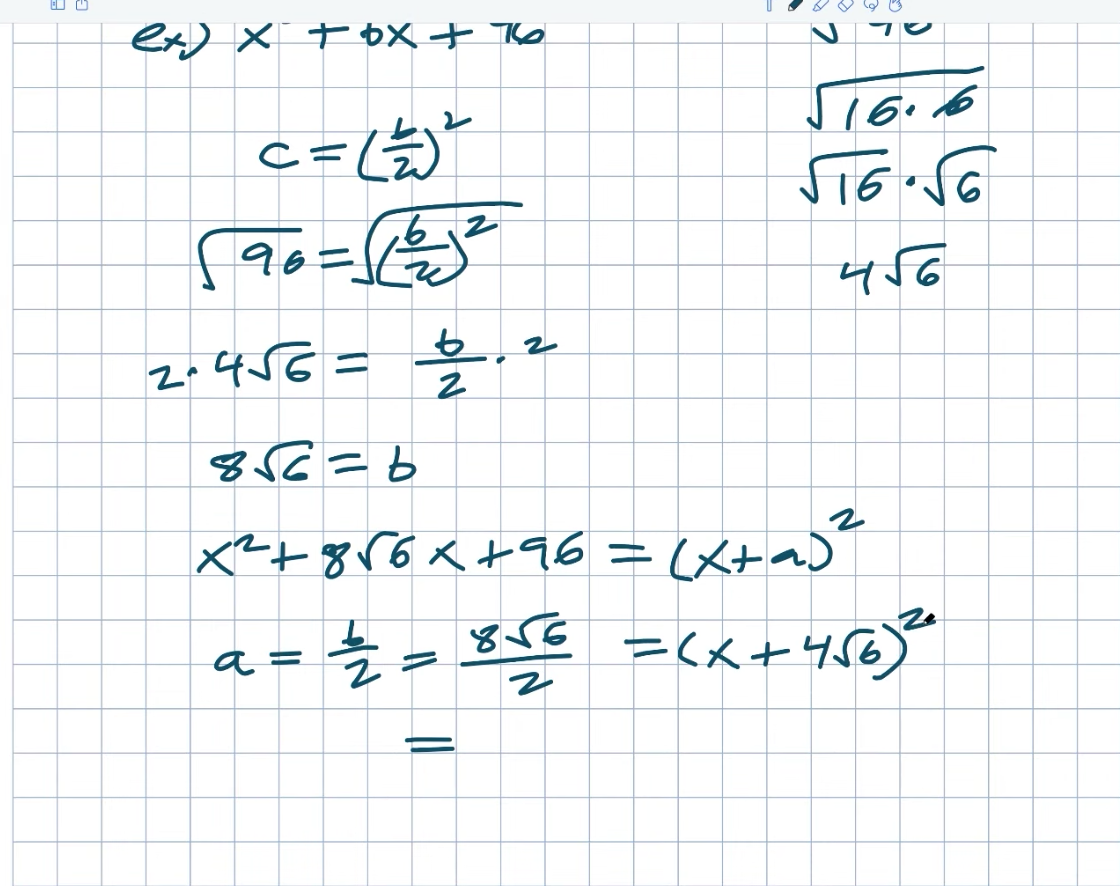As a result, I take the square root of both sides. The square root of 96 is now. We only recently discovered this. As a result, a four radical six equals a B divided by two. Multiply by two on both sides once more. As a result, I get an eight radical six equals a B. It appears to be a little perplexing. But, once again, that will be the answer we seek. So I have an X squared plus an eight square root of six, for a total of X plus 96. Let’s take a look at what my A is once more. Now. So, if I want to figure this out, I have an X. Let’s say that’s going to be a plus. As a result, plus a quantity squared. So, how would you simplify that? So, once again, what we needed to be able to do to find our A is simply my B divided by two. And, once again, we’ve already determined that my B divided by two equals a four square root of six. Right. Alternatively, you can simply multiply eight by six. Right. Remember that A equals B divided by two, which in this case is eight squared of six divided by two. As a result, it is equal to an X plus a four square root of six, multiplied by the quantity squared. And, once again, let’s double-check to make sure everything is in order. So four squared six times four squared six equals sixteen squared six, which equals 96, which is exactly what we’re looking for.

Now, in this video, I hope to develop a better understanding of our perfect square trinomials, binomial squared, and the process of identifying those values because that is the essence of completing the square. We didn’t actually get into problems of solving using completing the square, but that’s what I want to do in the next post, working through how to find the solutions of solving using completely in the square.